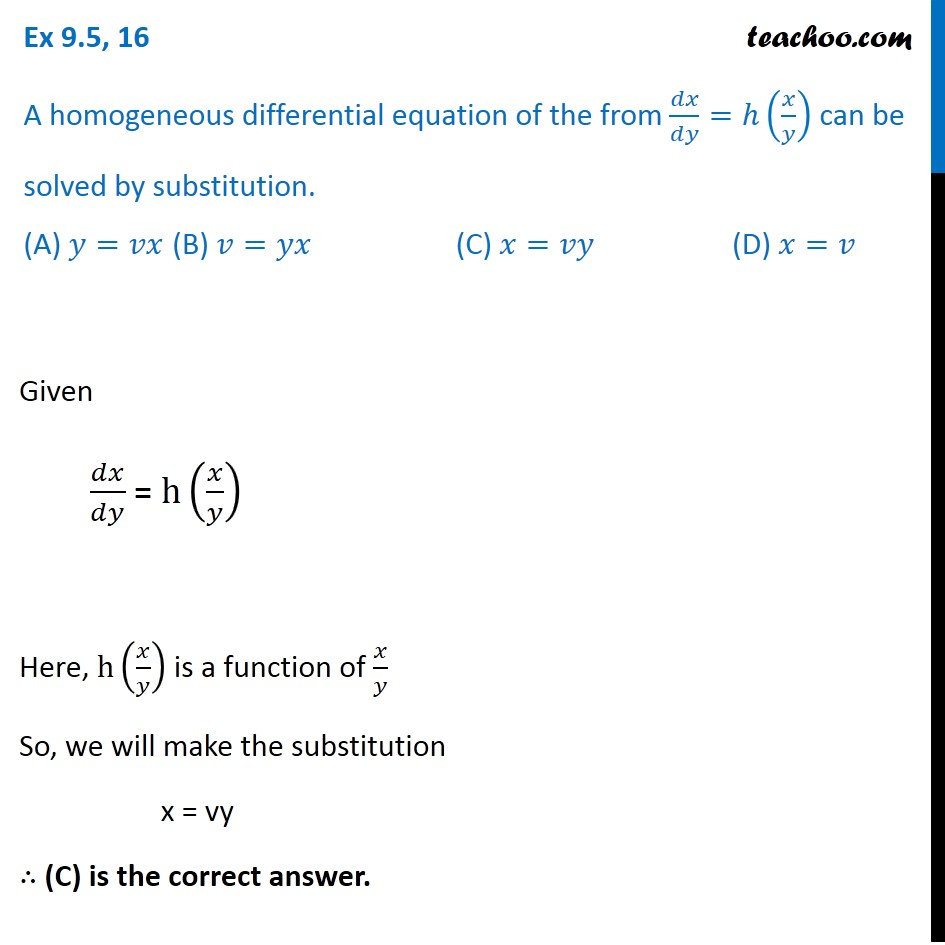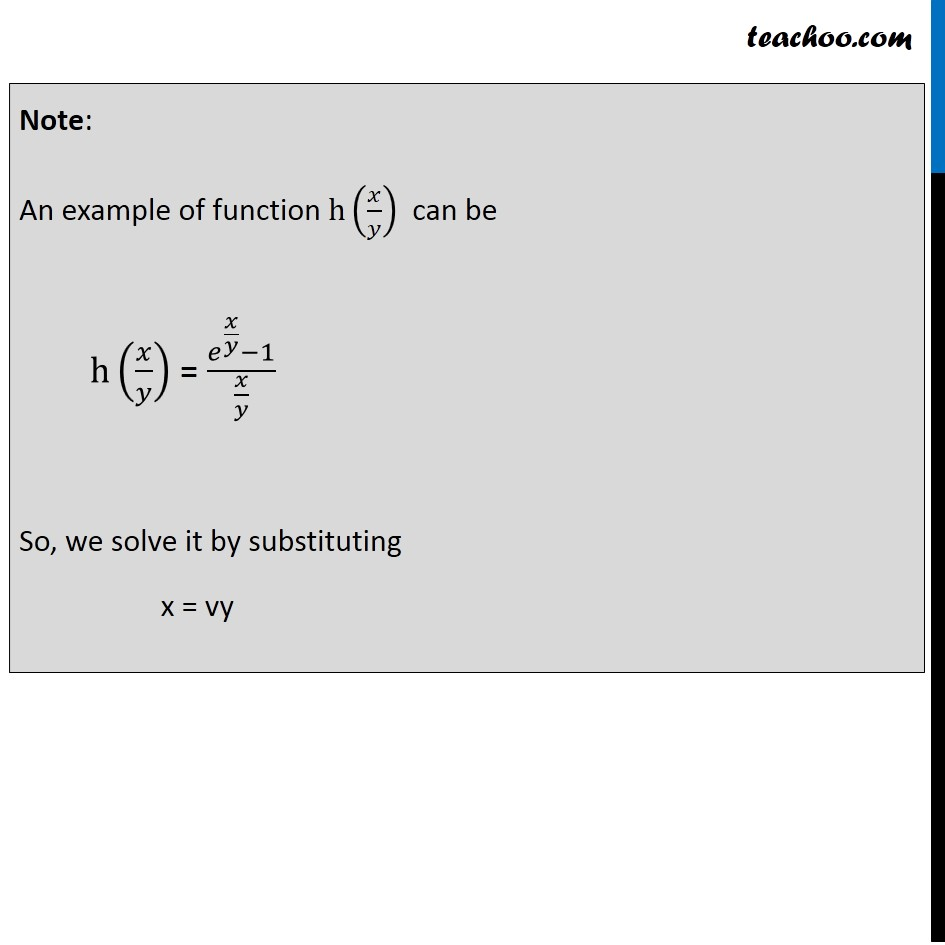Ex 9.4

Chapter 9 Class 12 Differential Equations
Serial order wiseLearn in your speed, with individual attention - Teachoo Maths 1-on-1 Class

### Transcript

Ex 9.4, 16 A homogeneous differential equation of the from 𝑑𝑥/𝑑𝑦=ℎ(𝑥/𝑦) can be solved by substitution. (A) 𝑦=𝑣𝑥 (B) 𝑣=𝑦𝑥 (C) 𝑥=𝑣𝑦 (D) 𝑥=𝑣 Given 𝑑𝑥/𝑑𝑦 = h(𝑥/𝑦) Here, h(𝑥/𝑦) is a function of 𝑥/𝑦 So, we will make the substitution x = vy ∴ (C) is the correct answer. Note: An example of function h(𝑥/𝑦) can be h(𝑥/𝑦) = (𝑒^(𝑥/𝑦)−1)/(𝑥/𝑦) So, we solve it by substituting x = vy